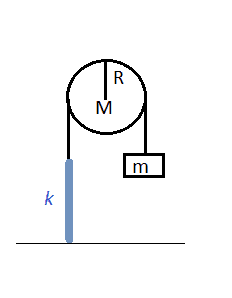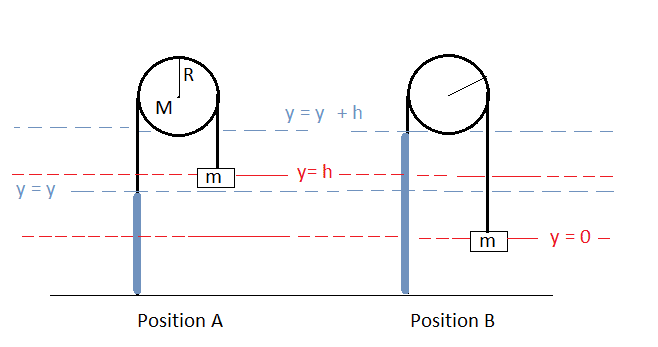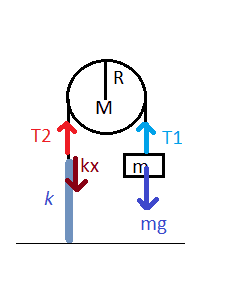# Spring - Pulley - Mass system. Proving omega is a certain value

• Anavra
In summary, the student is seeking help with a physics problem involving a block attached to a spring and pulley system. They have attempted to use conservation of energy and Newton's second law to solve for the angular frequency of oscillation but have not been successful. They are looking for hints and suggestions to help them solve the problem.

## Homework Statement

Hello, everyone. I am a starting physics student. I know a little bit of calculus and mechanics. I have been solving a lot of problems successfully, but I come back to being stuck on this one. I will show you what I have done and I hope you can be kind enough to give me a general hint, I would really appreciate it. Am I doing something really wrong? I've been going around for days.

THANKS!

So here it goes:

A block of mass m is attached to a vertical spring by a rope hanging over a pulley (I = 1/2 MR2). The pulley has a mass M and a radius R. The rope does not slip and does not stretch.

Show that the angular frequency of oscillation is ω = $\frac{2k}{M+m}$

It was accompanied by a drawing that looks like this.## Homework Equations

Conservation of mechanical energy.
Total Energy at position A = Total Energy at position B = E (constant)

Potential Elastic Energy = 1/2ky2
Potential Gravitational Energy = mgy

Kinetic Translational Energy = 1/2mv2
Kinetic Rotational Energy = 1/2 Iω2

v = ωR

Newton's second law
F = ma
τ = Iα = rxF

## The Attempt at a SolutionTotal Energy of Position A (As shown in picture)

E = 1/2ky2 + mgh + 1/2 mv2 + 1/2 Iω2
E = 1/2ky2 + mgh + 1/2 mv2 + 1/2 I (v2/R2)
E = 1/2ky2 + mgh + 1/2 mv2 + 1/4 MR2 (v2/R2)
E = 1/2ky2 + mgh + 1/2 mv2 + 1/4 Mv2
E = 1/2ky2 + mgh + (1/2 m + 1/4 M)v2

Total Energy of Position B (As shown in picture). I took position B as being the one with maximum amplitude, at which point the movement changes direction and so the velocities are 0, as are the kinetic energies. The little mass m is also at its designated y=0, so the only energy at this point is the energy of the stretched spring, and it's equal to the same constant.

E = 1/2k(y + h)2
E = 1/2k(y2 + 2yh + h2)

By conservation of energy.
1/2ky2 + mgh + (1/2 m + 1/4 M)v2 = 1/2k(y2 + 2yh + h2)
mgh + (1/2 m + 1/4 M)v2 = kyh + 1/2kh2
h(mg - ky) + (1/2 m + 1/4 M)v2 = + 1/2kh2

It doesn't seem like this is ever going to become the neat equation for omega above. I notice that the first term is a sum of forces, that could result from Newton's second Law, except that this is not accounting for the moment of inertia of the pulley, that would make the tensions different. But even if the term (mg - ky) were equal to ma, that still leaves me with a differential equation with an acceleration and a velocity that I don't really recognize (Simple harmonic movement usually ends with an equation with an acceleration and a displacement, from which I can extract the angular frequency.)

Here's the free body diagram that I made of the system.By Newton's second Law,

For the mass:
T1 - mg = ma

For the point between the rope and the spring, it's thought of as mass-less, so to stop it from having an infinite acceleration from any net force:

T2 = ky

Now the pulley, the net torque is given by:

τ = Iα = RxF
R(T1 - T2) = Iα
R(T1 - ky) = I $\ddot{θ}$
R(mg + ma - ky) = I $\ddot{θ}$

Now, a = αR, and y=θR

Rmg - R2m$\ddot{θ}$ - R2kθ = I $\ddot{θ}$
R(mg - Rkθ) = $\ddot{θ}$ (I + R2m)
R(mg - Rkθ) = $\ddot{θ}$ (1/2R2M+ R2m)

This equation does have an acceleration in terms of a displacement, so it looks better, but it hasn't taken me anywhere near the wanted result.

Any and all hints greatly appreciated. I'm completely stuck and beginning to hate this problem.

Thank you all.

Last edited:
Anavra said:
So here it goes:

A block of mass m is attached to a vertical spring by a rope hanging over a pulley (I = 1/2 MR2). The pulley has a mass M and a radius R. The rope does not slip and does not stretch.

Show that the angular frequency of oscillation is ω = $\frac{2k}{M+m}$By Newton's second Law,

For the mass:
T1 - mg = ma

For the point between the rope and the spring, it's thought of as mass-less, so to stop it from having an infinite acceleration from any net force:

T2 = ky

Now the pulley, the net torque is given by:

τ = Iα = RxF
R(T1 - T2) = Iα
R(T1 - ky) = I $\ddot{θ}$
R(mg + ma - ky) = I $\ddot{θ}$

Now, a = αR, and y=θR

Rmg - R2m$\ddot{θ}$ - R2kθ = I $\ddot{θ}$
R(mg - Rkθ) = $\ddot{θ}$ (I + R2m)
R(mg - Rkθ) = $\ddot{θ}$ (1/2R2M+ R2m)

This equation does have an acceleration in terms of a displacement, so it looks better, but it hasn't taken me anywhere near the wanted result.

Any and all hints greatly appreciated. I'm completely stuck and beginning to hate this problem.

Thank you all.

The equation is that of a harmonic oscillator, with a constant force applied also on it. You can simplify by R and it would look simpler with a variable of length dimension x=Rθ. Try the solution in the form x=Asin(ωt)+B. The angular frequency you quoted is not correct, however.

ehild